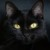# EXERCISE 11.11. A square and a rectangular field withmeasurements as given in the figure have the sameperimeter.

EXERCISE 11.1
1. A square and a rectangular field with
measurements as given in the figure have the same
perimeter. Which field has a larger area?​

### 1 thought on “EXERCISE 11.1<br />1. A square and a rectangular field with<br />measurements as given in the figure have the same<br />perimeter.”

1.The side of a square = 60 m

And the length of rectangular field = 80 m

According to question,

Perimeter of rectangular field

= Perimeter of square field

= 4 side

m

Now Area of Square field

=

= = 3600 m2

And Area of Rectangular field

= length breadth = 80 40

= 3200

Hence, area of square field is larger